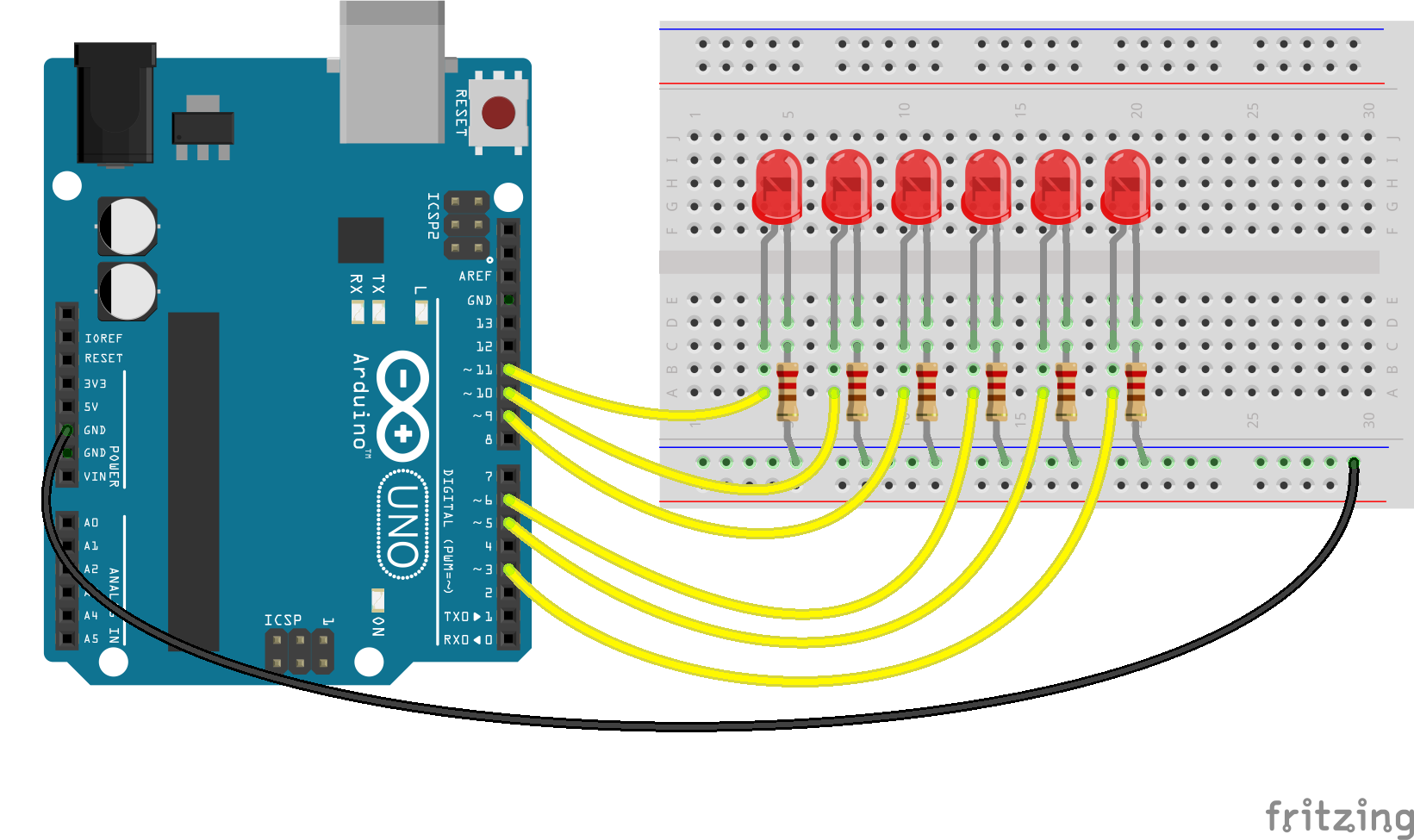Demonstrates use of callbacks to fade out a series of LEDs one after the other. Requires LEDs on pins that support PWM (usually denoted by ~).Run this example from the command line with:

``````node eg/led-fade-callback.js
``````
``````const {Board, Leds} = require("johnny-five");
const board = new Board();

// Set up the following PWM pins as LEDs.
// Fade an LED out, and the complete callback will start
// fading the next LED in sequence out, and so on.
const leds = new Leds([11, 10, 9, 6, 5, 3]);
const timing = 250;
const ledCount = leds.length;

// Determine the next LED to fade
candidateIndex = Math.round(Math.random() * (ledCount - 1));
}
} else {
candidateIndex = (fadeIndex < ledCount - 1) ? fadeIndex + 1 : 0;
}

}

leds.on();